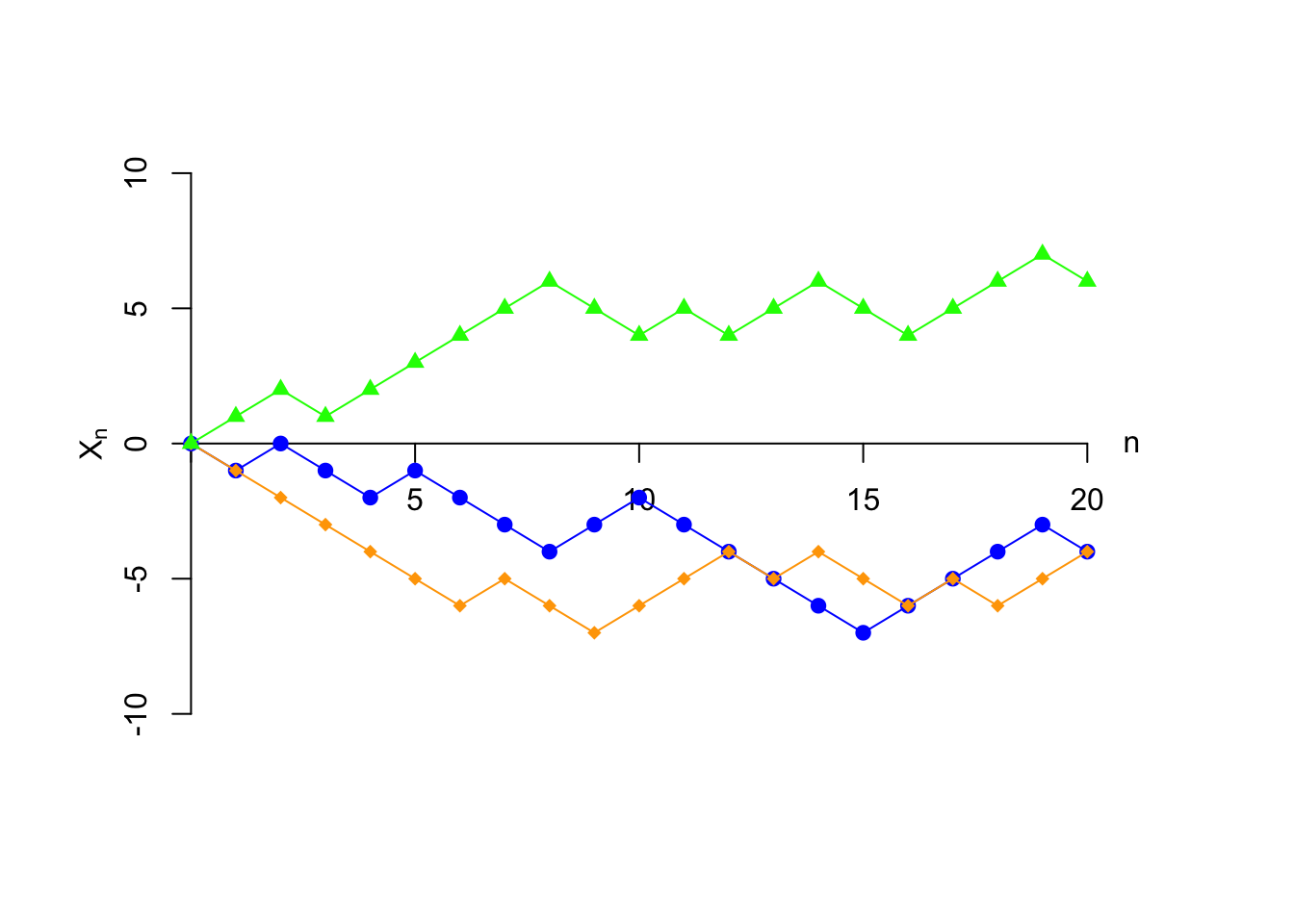# Lesson 31 Random Walk

## Theory

The random walk (also known as the “drunkard’s walk”) is an example of a random process evolving over time, like the Poisson process (Lesson 17).

The setup for the random walk is as follows. A drunk man is stumbling home from a bar. Because of his inebriated state, each step he takes is equally likely to be one step forward or one step backward, independent of any other step.

In other words, the $$i$$th step is a random variable $$Z_i$$, with p.m.f.

$\begin{array}{r|cc} z & -1 & 1 \\ \hline f(z) & 0.5 & 0.5 \end{array}$

and the $$Z_i$$s are independent.

The drunkard’s position after $$n$$ steps is $X_n = Z_1 + Z_2 + \ldots + Z_n.$ (We assume that he starts at the origin $$X_0 = 0$$.)

Shown below are three possible realizations of the random walk. A realization of a random process over time is called a sample path. We plot the three sample paths below, each of which shows the position of the drunkard $$X_n$$ as a function of $$n$$. Although all three sample paths start at 0 at $$n=0$$, they diverge very quickly.## Essential Practice

Answer the following questions about the random walk. You should use linearity of expectation and properties of covariance as much as possible.

1. What is the distribution of $$X_2$$, the drunkard’s position after just $$2$$ steps? (This is not a named distribution. Just make a table showing the possible values and their probabilities.)
2. Calculate $$E[Z_i]$$ and $$\text{Var}[Z_i]$$.
3. Calculate $$E[X_3]$$ and $$\text{Var}[X_3]$$.
4. Consider $$X_3$$ and $$X_5$$. Do you think their covariance is positive, negative, or zero? Calculate $$\text{Cov}[X_3, X_5]$$.